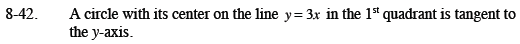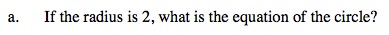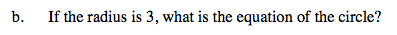Home > CCA2 > Chapter 8 > Lesson 8.1.2 > Problem8-42

8-42.
1. A circle with its center on the line y = 3x in the 1st quadrant is tangent to the y-axis. 8-42 HW eTool (Desmos). Homework Help ✎

1. If the radius is 2, what is the equation of the circle?

2. If the radius is 3, what is the equation of the circle?What point on the line y = 3x is 2 units from the y-axis?

Use the coordinates of the center and the radius to write an equation in general form.See part (a).

Use the eTool below for help with this problem.
Click on the link at right for the full eTool version: CCA2 8-42 HW eTool